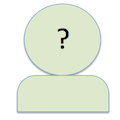cpollett

Dec 6 In-Class Exercise Thread. - 06/12/2017CS 256 Fall 2017

Post to this thread your solutions to the Dec 6 In-Class Exercise.
Best,
Chris
Post to this thread your solutions to the Dec 6 In-Class Exercise. Best, Chrisvasuvenk

-- Dec 6 In-Class Exercise Thread. - 06/12/2017CS 256 Fall 2017

((resource:pic.jpeg|Resource Description for pic.jpeg))ctmackay

-- Dec 6 In-Class Exercise Thread. - 06/12/2017CS 256 Fall 2017

calculate Xbar. (each pixel has a value of <1,0,0> or <0,1,0> or <0,0,1>. sum these up, and divide by 1/(3*r*c) = 9/27= 1/3
calculate std dev. the 3rd term will always evaluate to (1-1/3)^2+ (-1/3)^2 + (-1/3)^2 no matter which pixel we are looking at = 2/3.
multiplied by number of cells 9, divided by 1/(3*r*c) = (2/3)*9 * 1/(3*3*3) = 2/9
constrast = sqrt(2/9)
both images are the same, because each pixel still has the form of <1,0,0>. THe contrast is a measure between the brightest and darkest pixels. which in the two images are the same.

Last Edited: 06/12/2017
calculate Xbar. (each pixel has a value of <1,0,0> or <0,1,0> or <0,0,1>. sum these up, and divide by 1/(3*r*c) = 9/27= 1/3 calculate std dev. the 3rd term will always evaluate to (1-1/3)^2+ (-1/3)^2 + (-1/3)^2 no matter which pixel we are looking at = 2/3. multiplied by number of cells 9, divided by 1/(3*r*c) = (2/3)*9 * 1/(3*3*3) = 2/9 constrast = sqrt(2/9) both images are the same, because each pixel still has the form of <1,0,0>. THe contrast is a measure between the brightest and darkest pixels. which in the two images are the same.goutham

-- Dec 6 In-Class Exercise Thread. - 06/12/2017CS 256 Fall 2017

((resource:assign.jpg|Resource Description for assign.jpg))abhinavvarma123

-- Dec 6 In-Class Exercise Thread. - 06/12/2017CS 256 Fall 2017

((resource:cs256.jpeg|Resource Description for cs256.jpeg))nishanth

-- Dec 6 In-Class Exercise Thread. - 06/12/2017CS 256 Fall 2017

((resource:WhatsApp Image 2017-12-06 at 5.24.43 PM.jpeg|Resource Description for WhatsApp Image 2017-12-06 at 5.24.43 PM.jpeg))abhi

-- Dec 6 In-Class Exercise Thread. - 06/12/2017CS 256 Fall 2017

((resource:WhatsApp Image 2017-12-06 at 5.42.59 PM.jpeg|Resource Description for WhatsApp Image 2017-12-06 at 5.42.59 PM.jpeg))ishan50492

-- Dec 6 In-Class Exercise Thread. - 06/12/2017CS 256 Fall 2017

((resource:IMG_7773.JPG|Resource Description for IMG_7773.JPG))nmauthes

-- Dec 6 In-Class Exercise Thread. - 06/12/2017CS 256 Fall 2017

Avg. = 1/3
In both cases the contrast is 2/(3*sqrt(3))
Avg. = 1/3 In both cases the contrast is 2/(3*sqrt(3))jason.chee

-- Dec 6 In-Class Exercise Thread. - 06/12/2017CS 256 Fall 2017

r=3, c=3
1/(3rc) = 1/27
x_bar = 1/27 * 9 = 1/3
contrast of image = sqrt(1/27 * [3(1-1/3)^2+ 3(1-1/3)^2+ 3(1-1/3)^2]) = sqrt(1/3 * 4/9)
=2/(3*sqrt(3))
r=3, c=3 1/(3rc) = 1/27 x_bar = 1/27 * 9 = 1/3 contrast of image = sqrt(1/27 * [3(1-1/3)^2+ 3(1-1/3)^2+ 3(1-1/3)^2]) = sqrt(1/3 * 4/9) =2/(3*sqrt(3))

[X ]